# (1 point) Solve the initial value problem dx -H x(0) х, dt Give your solution in...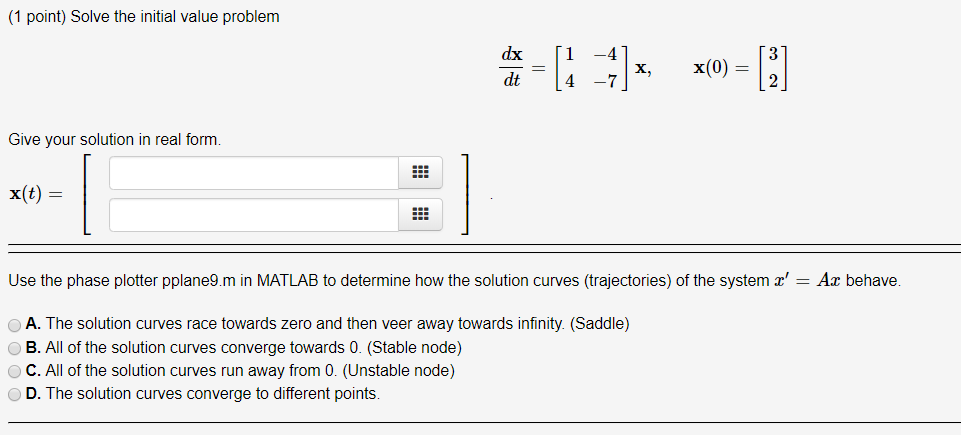(1 point) Solve the initial value problem dx -H x(0) х, dt Give your solution in real form. x(t) Use the phase plotter pplane9.m in MATLAB to determine how the solution curves (trajectories) of the system x' = Ax behave. A. The solution curves race towards zero and then veer away towards infinity. (Saddle) B. All of the solution curves converge towards 0. (Stable node) C. All of the solution curves run away from 0. (Unstable node) D. The solution curves converge to different points.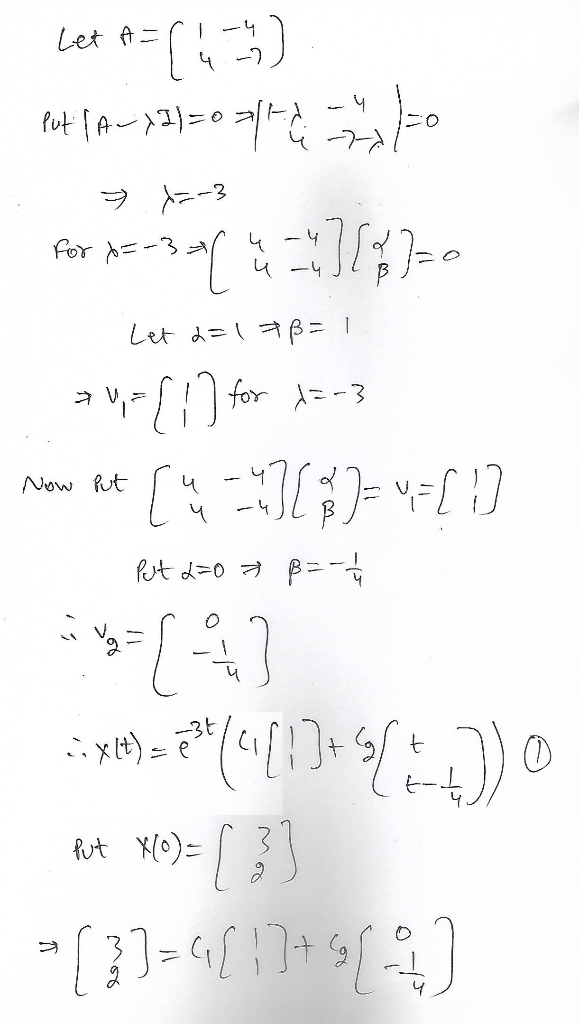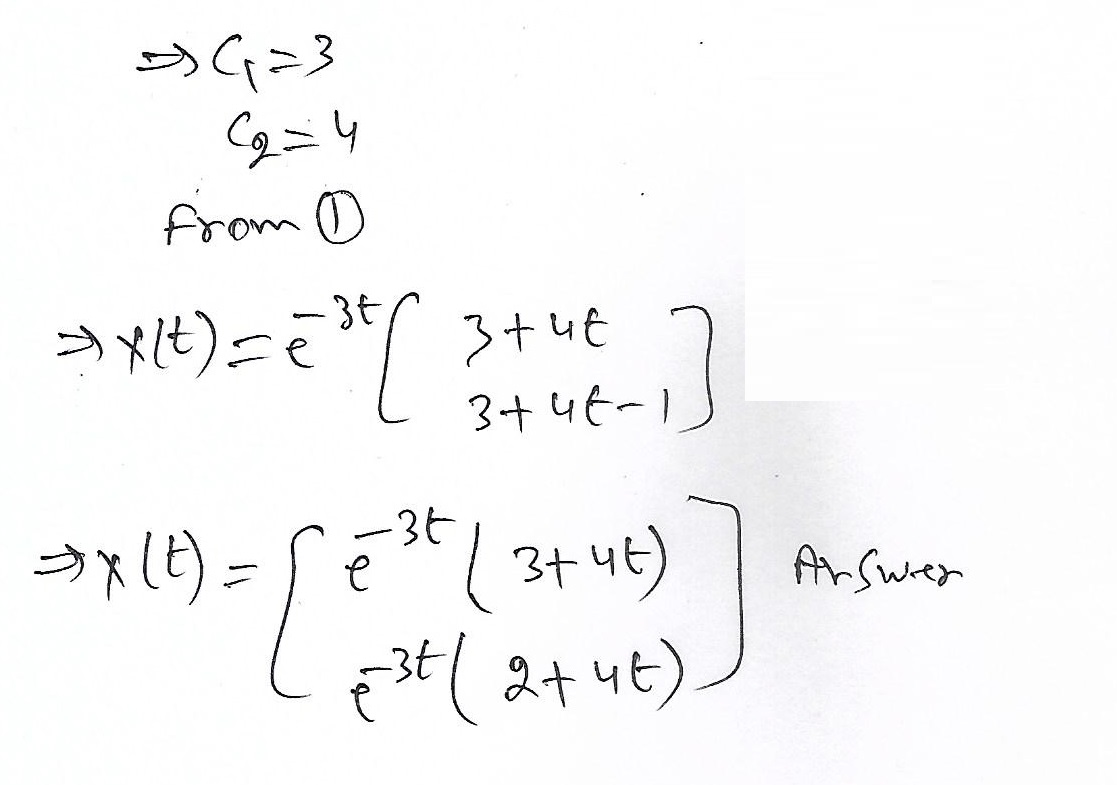#### Earn Coin

Coins can be redeemed for fabulous gifts.

Similar Homework Help Questions
• ### (1 point) Solve the initial value problem dx 1.5 2. -1.5 1,5) X, x(0) = (-3)...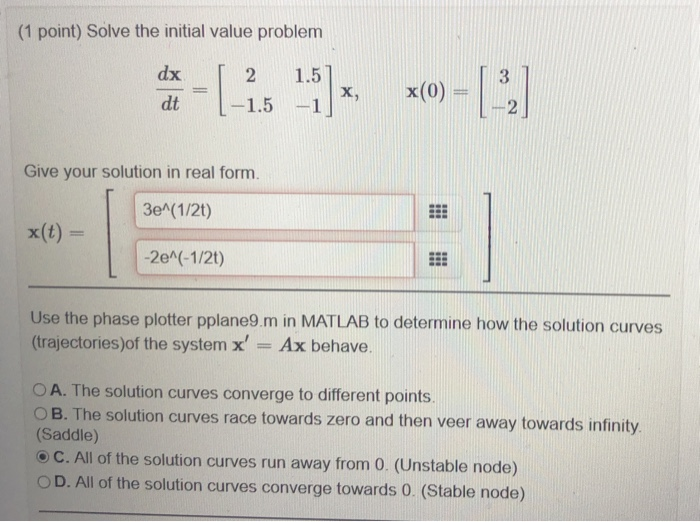(1 point) Solve the initial value problem dx 1.5 2. -1.5 1,5) X, x(0) = (-3) dt -1 Give your solution in real form. 3e^(1/2) x(t) = -2e^(-1/2t) Use the phase plotter pplane9.m in MATLAB to determine how the solution curves (trajectories)of the system x' Ax behave. O A. The solution curves converge to different points. OB. The solution curves race towards zero and then veer away towards infinity (Saddle) C. All of the solution curves run away from 0....

• ### (1 point) Consider the system of differential equations dx dt = -1.6x + 0.5y, dy dt...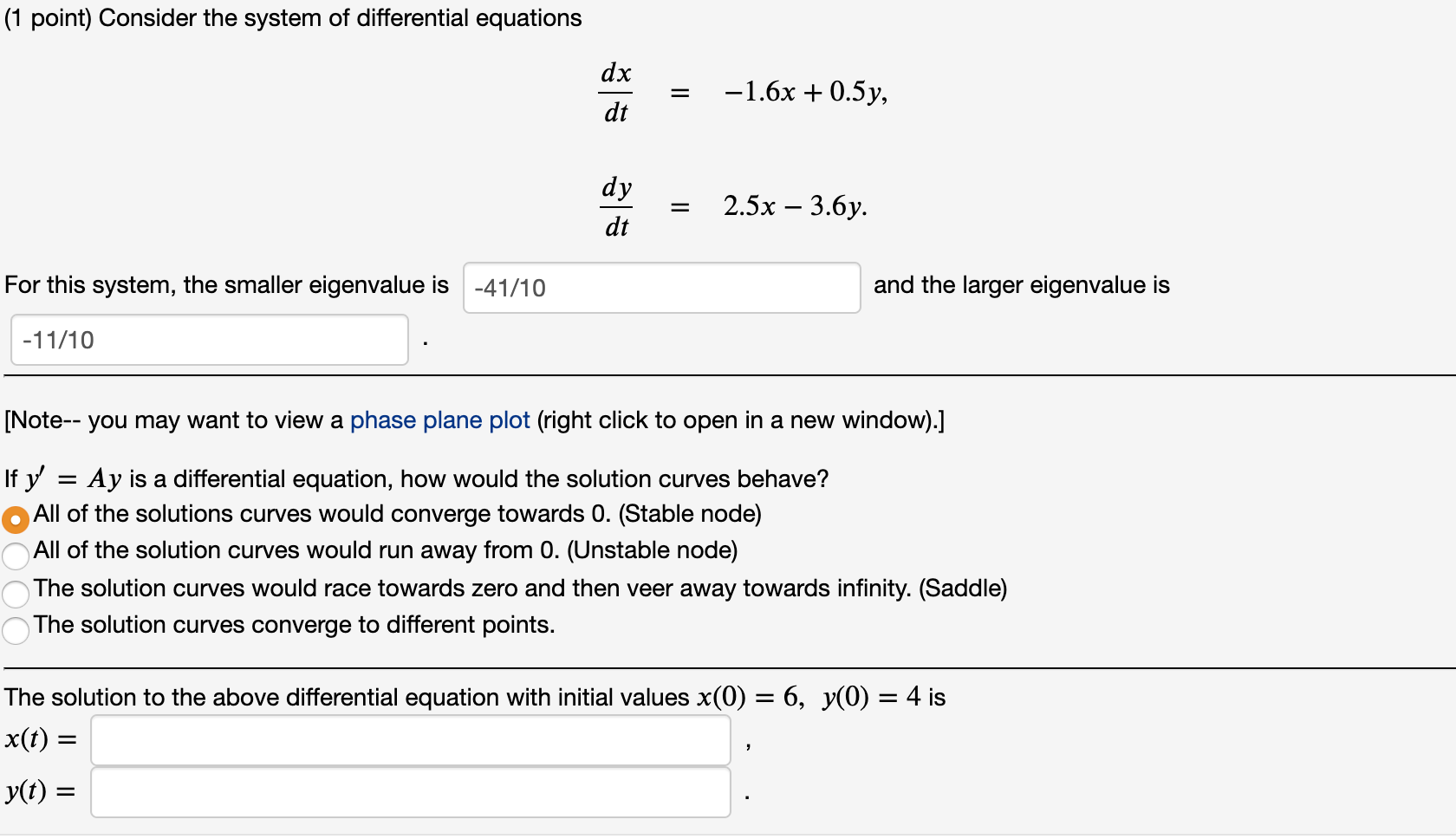(1 point) Consider the system of differential equations dx dt = -1.6x + 0.5y, dy dt = 2.5x – 3.6y. For this system, the smaller eigenvalue is -41/10 and the larger eigenvalue is -11/10 [Note-- you may want to view a phase plane plot (right click to open in a new window).] If y' Ay is a differential equation, how would the solution curves behave? All of the solutions curves would converge towards 0. (Stable node) All of the solution...

• ### (1 point) a. Find the most general real-valued solution to the linear system of differential equations...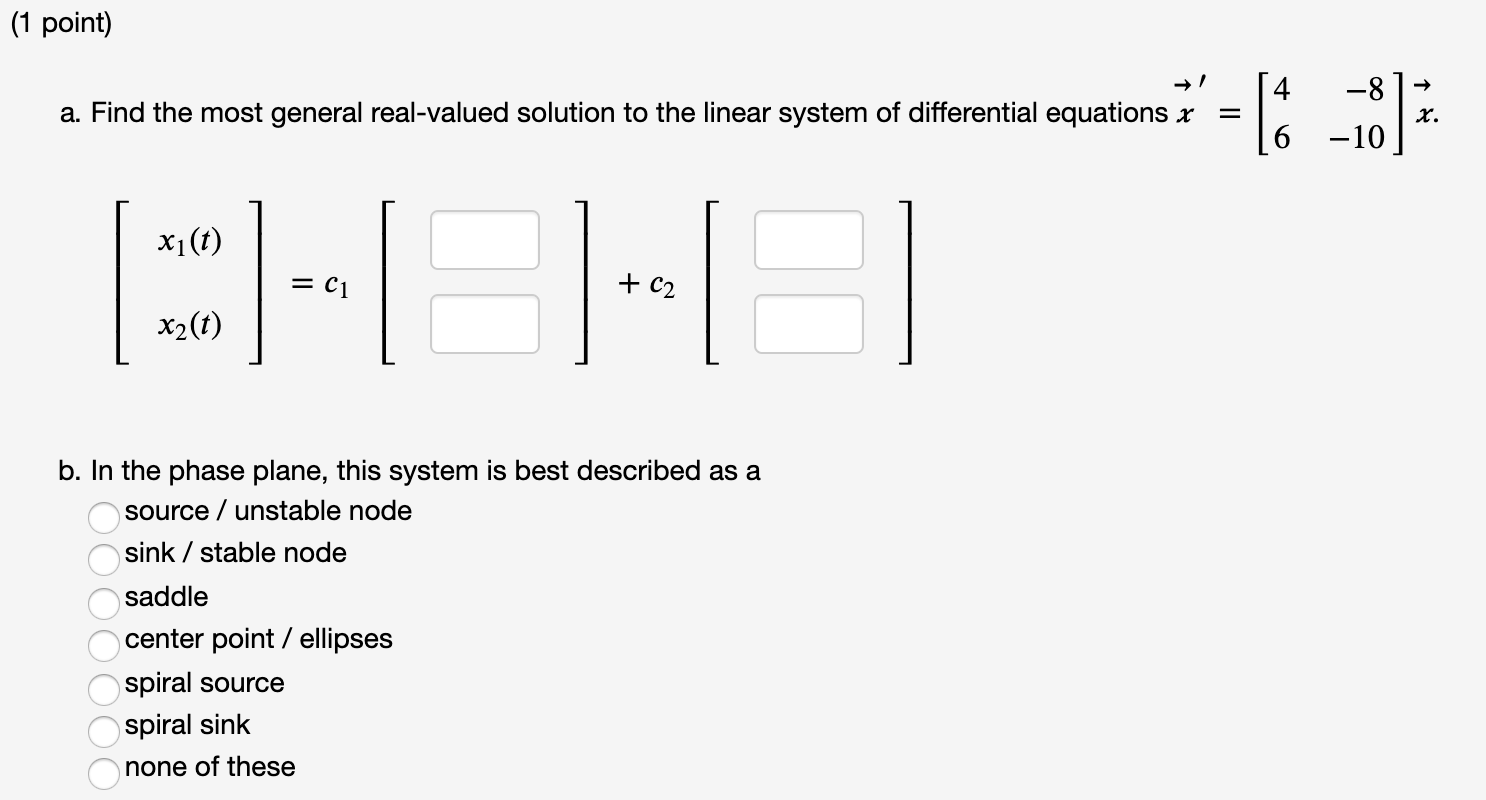(1 point) a. Find the most general real-valued solution to the linear system of differential equations x -8 -10 x. xi(t) = C1 + C2 x2(t) b. In the phase plane, this system is best described as a source / unstable node sink / stable node saddle center point / ellipses spiral source spiral sink none of these ОООООО (1 point) Calculate the eigenvalues of this matrix: [Note-- you'll probably want to use a calculator or computer to estimate the...

• ### = 3x +0.75y, = 1.66667x + y. For this system, the smaller eigenvalue is 1/2 and...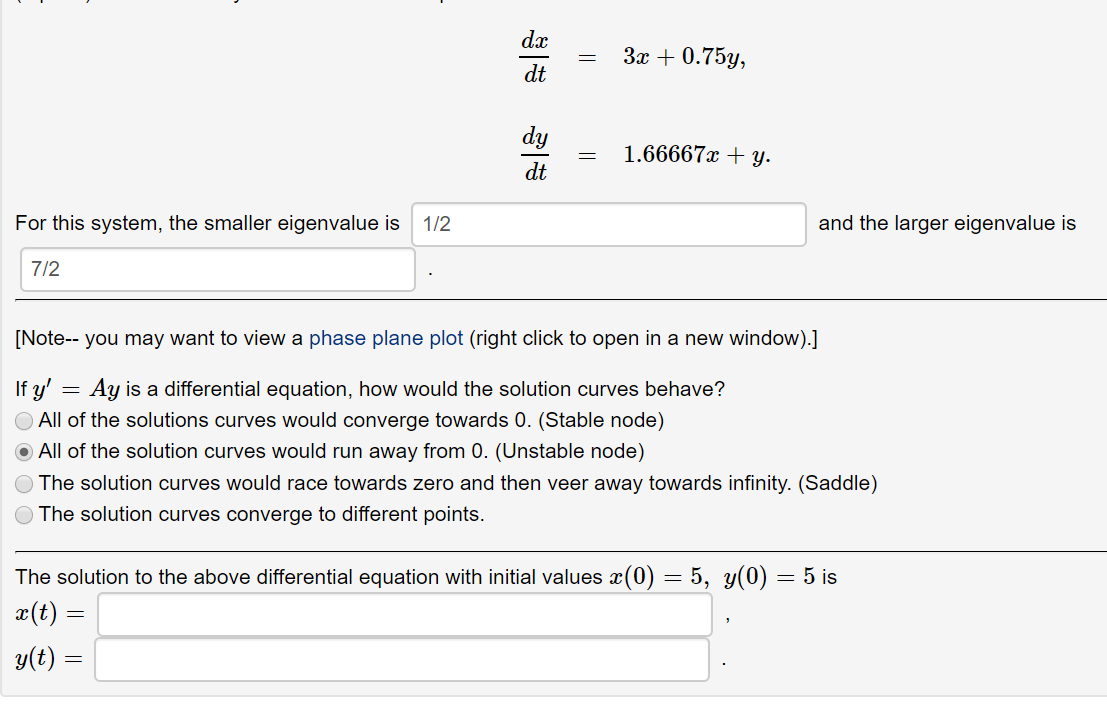= 3x +0.75y, = 1.66667x + y. For this system, the smaller eigenvalue is 1/2 and the larger eigenvalue is 7/2 [Note-- you may want to view a phase plane plot (right click to open in a new window).] If y' = Ay is a differential equation, how would the solution curves behave? All of the solutions curves would converge towards 0. (Stable node) All of the solution curves would run away from 0. (Unstable node) The solution curves would...

• ### (1 point) Given that ū = and are eigenvectors of the matrix -12 24 determine the...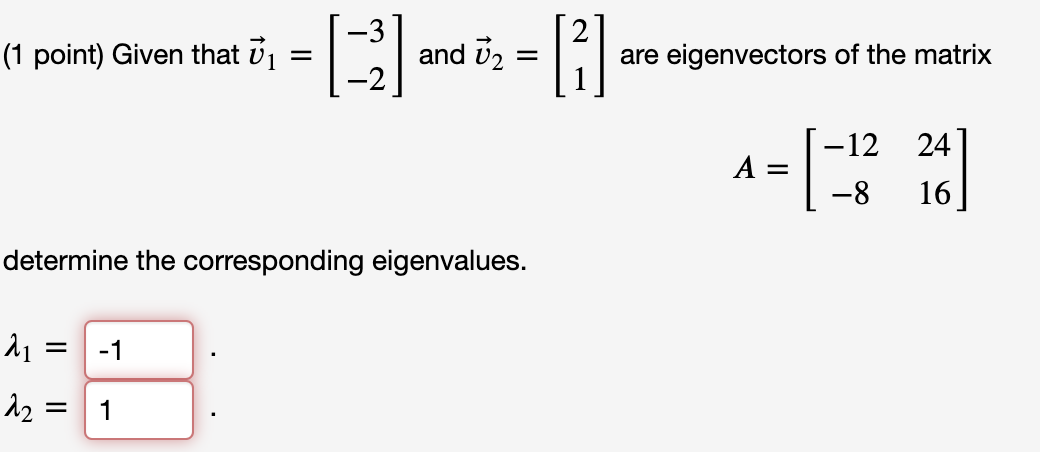(1 point) Given that ū = and are eigenvectors of the matrix -12 24 determine the corresponding eigenvalues. 21 = -1 12 = 1 (1 point) Solve the system -6 1 dx dt х -6 -1 with the initial value 0 x(0) = -2 x(t) = (1 point) Calculate the eigenvalues of this matrix: [Note-- you'll probably want to use a calculator or computer to estimate the roots of the polynomial which defines the eigenvalues. You also may want to...

• ### (1 point) Calculate the eigenvalues of this matrix: [Note-- you'll probably want to use a calculator...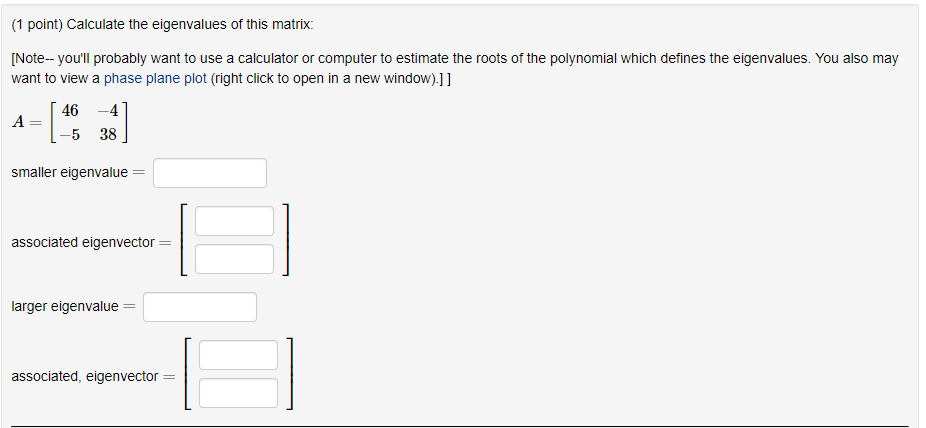(1 point) Calculate the eigenvalues of this matrix: [Note-- you'll probably want to use a calculator or computer to estimate the roots of the polynomial which defines the eigenvalues. You also may want to view a phase plane plot (right click to open in a new window).]] 46 A -4 38 -5 smaller eigenvalue associated eigenvector = larger eigenvalue associated, eigenvector (1 point) Consider the system of differential equations dr dt 3x + 0.5y, dy 2.5x + y. dt For...

• ### (1 point) Consider the Initial Value Problem -5 dx dt X x(0) (a) Find the eigenvalues...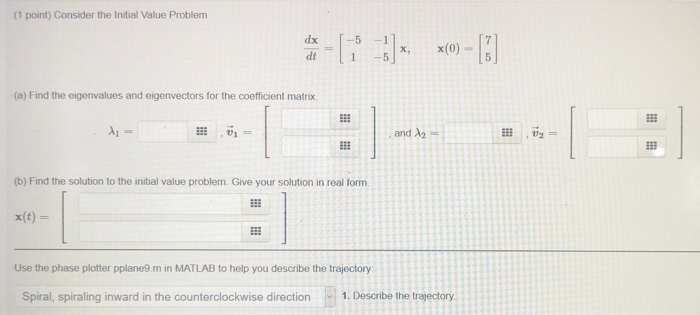(1 point) Consider the Initial Value Problem -5 dx dt X x(0) (a) Find the eigenvalues and eigenvectors for the coefficient matrix A = and 2 -- 1 333 (b) Find the solution to the initial value problem. Give your solution in real form Use the phase plotter pplane9.m in MATLAB to help you describe the trajectory Spiral, spiraling inward in the counterclockwise direction 1. Describe the trajectory

• ### Problem 3. Consider the following continuous differential equation dx dt = αx − 2xy dy dt...

Problem 3. Consider the following continuous differential equation dx dt = αx − 2xy dy dt = 3xy − y 3a (5 pts): Find the steady states of the system. 3b (15 pts): Linearize the model about each of the fixed points and determine the type of stability. 3b (15 pts): Draw the phase portrait for this system, including nullclines, flow trajectories, and all fixed points. Problem 2 (25 pts): Two-dimensional linear ODEs For the following linear systems, identify the...

• ### The equation for the unforced, damped pendulum is 2 d0 -mg sin ml 2 dt which, calling 0 by x, can be written as a pair of first order ODE's dx 2 dt For Quiz 9, please submit the phase plane for e...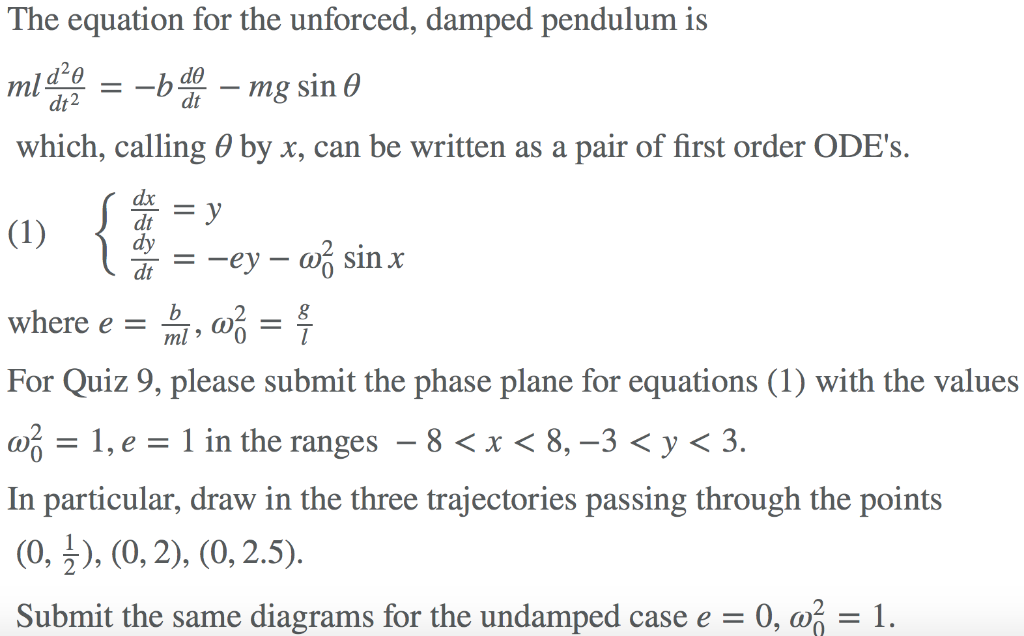The equation for the unforced, damped pendulum is 2 d0 -mg sin ml 2 dt which, calling 0 by x, can be written as a pair of first order ODE's dx 2 dt For Quiz 9, please submit the phase plane for equations (1) with the values -1,e1 in the ranges -8 < x < 8,-3< y < 3. 0 In particular, draw in the three trajectories passing through the points Submit the same diagrams for the undamped case e...